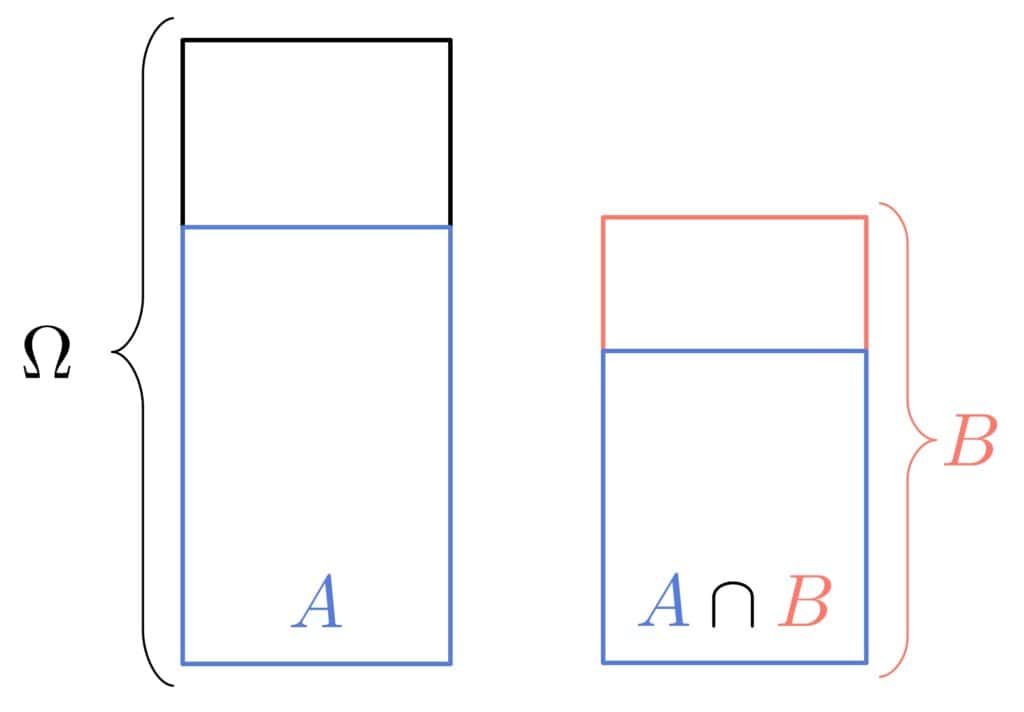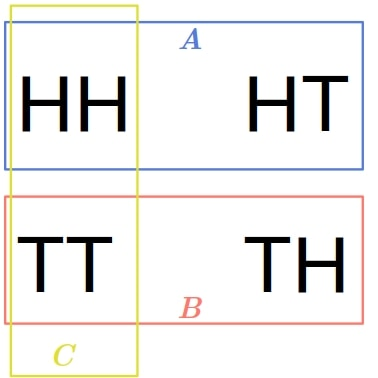## 1. Introduction

In this tutorial, we’ll explain statistical independence.

## 2. Why Is the Independence of Events Important?

Merriam-Webster lists several meanings of the word independent. In mathematics, we usually go with a variation of “not determined by or capable of being deduced or derived from or expressed in terms of members (such as axioms or equations) of the set under consideration“.

Something similar holds in probability theory and statistics. We say that two events are statistically independent if the probability of one doesn’t change if we learn that the other event got realized (or didn’t occur) and vice versa.

But why do we bother with this?

Let’s say we’re developing a vaccine and want to check if an expensive component improves the protection. We need to test the vaccine on two randomly collected groups of test subjects. One group gets the expensive version, and the other receives the version without the component. If the incidence of the disease is the same in both groups, the chance that the vaccine protects against it won’t change if we add the component.

So, the events “the vaccine contains this very expensive component” and “the vaccine protects those who get a shot” will be independent. Consequently, we can produce the vaccine without an expensive part and save money.

## 3. Statistical Independence of Two Events

The independence of events has a precisely defined meaning in statistics. Eventsandare independent of one another (which we write as) if their joint probability is the product of their individual probabilities:

(1)For instance, ifand, the joint probabilityneeds to be 0.35 forandto be independent.

### 3.1. Conditional Interpretation

We can make independence more intuitive by using conditional probabilities. Let’s recall that:So, ifandare mutually independent, we get:From there:Therefore, if, the realization ofdoesn’t change the probability thatwill happen and vice versa.

For instance, if we flip a coin and get a head, the chances for the second toss to result in either side remain unaffected by the first outcome.

### 3.2. Visualization

Let’s visualize the probabilities in question as surfaces. If, the proportion the surface thattakes in the area ofshould be the same as the proportiontakes in the entire event space:## 4. Statistical Independence of Multiple Events

There are two notions of independence for multiple events.

### 4.1. Pairwise Independence

We say that eventsare pairwise independent if any two eventsare independent of one another in the sense of Equation (1}:

(2)However, that doesn’t mean that the intersections of three and more events decompose to the individual events’ probabilities.

Here’s a classical example illustrating this. If we toss a coin two times, we have four possible outcomes:. Let’s define three events:

•: the first toss gives us a head
•: the first toss yields a tail
•: the same side appears both times

Visually:Assuming that the coin is fair, all the outcomes are equally likely, so.

Further:As we see, the events are pairwise independent. However:So,isn’t independent of,of, andof. That’s what we have mutual independence for.

### 4.2. Mutually Independent Events

Eventsare mutually independent if any event is independent of any intersection of other events from the set.

So, we want the following to hold for anyandsuch thatandfor any:But, this also needs to hold for all the events and intersections in. So, we can write the condition compactly as:

(3)Therefore, mutually independent events are also pairwise independent.

## 5. Conditional Independence

Since conditional probability is also a probability, there’s also conditional independence:

(4)If the above holds for events,, and, we say thatandare conditionally independent given.

Intuitively, that means that ifhappened, the realization ofdoesn’t affect the chance ofoccurring. Or, in line with Bayesianism, any information onreveals nothing aboutprovided that we knowhas happened.

## 6. Independence of Random Variables

We can use the above notions of independence to define the independence of random variables. Since the same reasoning holds for pairwise and mutually independent random variables, we’ll focus on the independence of only two variables.

Variablesandare stochastically independent if the eventsandare independent for anyand.

Ifanddenote the variables’ cumulative distribution functions, andtheir joint CDF, the definition comes down to:

(5)## 7. Statistical vs. Everyday-Language Independence

The notions of independence in everyday language differ from those in probability and statistics.

Non-statisticians and non-mathematicians usually understand the independence of eventsandto mean they are completely unrelated. For example, when flipping a coin two times, people could say that the two outcomes aren’t independent because the same coin is used both times.

In contrast, a statistician would argue that the outcome of one flip doesn’t affect the realization of the other. So they would consider the two events statistically independent.

## 8. Conclusion

In this article, we explained statistical independence or events and random variables.